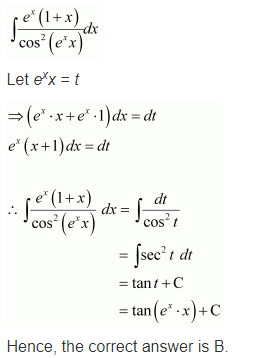ncert textbook

# NCERT Solutions for Class 12 Maths Chapter 7 Integers Ex 7.3

Get Free NCERT Solutions for Class 12 Maths Chapter 7 Integers Ex 7.3 PDF. Integration Class 12 Maths NCERT Solutions are extremely helpful while doing your homework. Exercise 7.3 Class 12 Maths NCERT Solutions were prepared by Experienced LearnCBSE.in Teachers. Detailed answers of all the questions in Chapter 7 Maths Class 12 Integrals Exercise 7.3 provided in NCERT Textbook.

The topics and sub-topics included in the Integrals chapter are the following:

 Section Name Topic Name 7 Integrals 7.1 Introduction 7.2 Integration as an Inverse Process of Differentiation 7.3 Methods of Integration 7.4 Integrals of some Particular Functions 7.5 Integration by Partial Fractions 7.6 Integration by Parts 7.7 Definite Integral 7.8 Fundamental Theorem of Calculus 7.9 Evaluation of Definite Integrals by Substitution 7.10 Some Properties of Definite Integrals

## NCERT Solutions for Class 12 Maths Chapter 7 Integers Ex 7.3

NCERT Solutions for Class 12 Maths Chapter 7 Integers Ex 7.3 are part of NCERT Solutions for Class 12 Maths. Here we have given Class 12 Maths NCERT Solutions Integrals Ex 7.3

NCERT Solutions for Class 12 Maths Chapter 7 Ex 7.3

Find the integrals of the functions in Exercises 1 to 22.
Question 1.
sin²(2x+5)
Solution: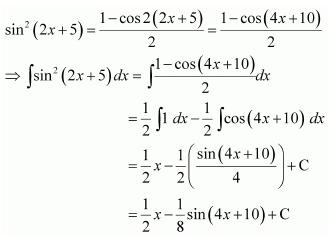Question 2.
sin3x cos4x
Solution: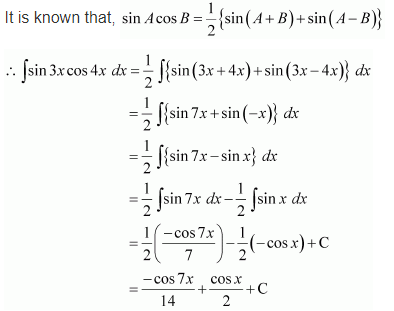Question 3.
∫cos2x cos4x cos6x dx
Solution: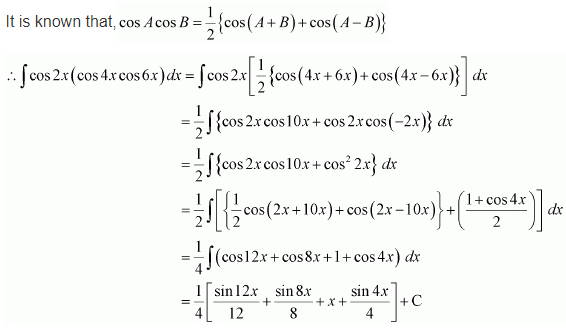Question 4.
∫sin3(2x+1)dx
Solution: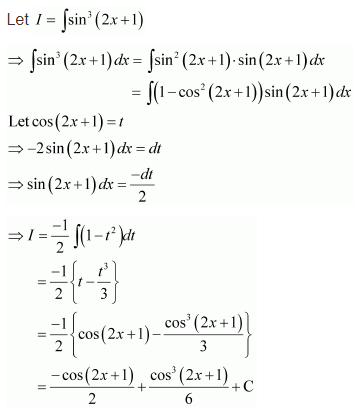Question 5.
sin3x cos3x
Solution: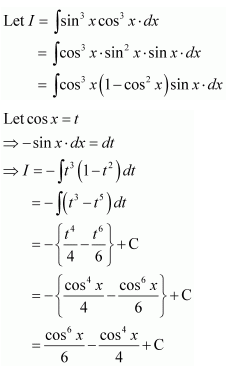Question 6.
sinx sin2x sin3x
Solution: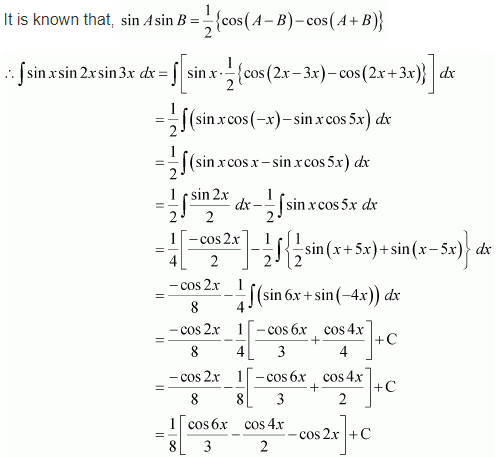Question 7.
sin 4x sin 8x
Solution: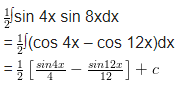Question 8.$\frac { 1-cosx }{ 1+cosx }$
Solution: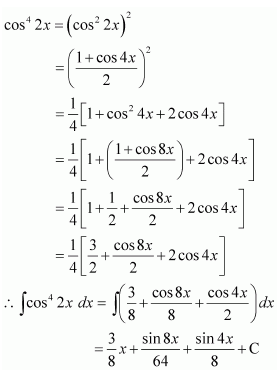Question 9.$\frac { cosx }{ 1+cosx }$
Solution: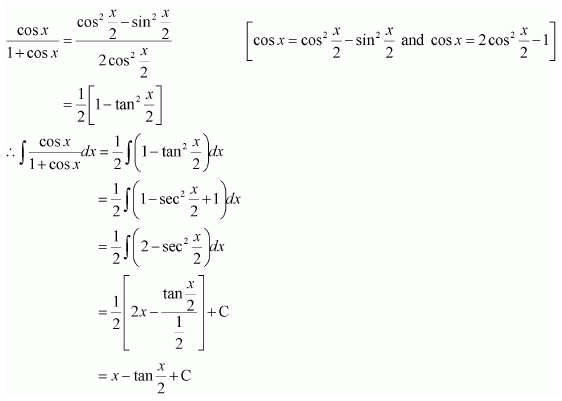Question 10.
∫sinx4 dx
Solution: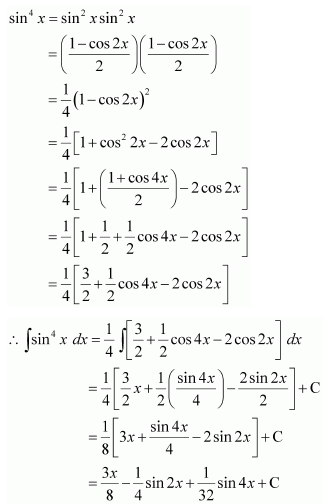Question 11.
cos4 2x
Solution:Question 12.$\frac { { sin }^{ 2 }x }{ 1+cosx }$
Solution: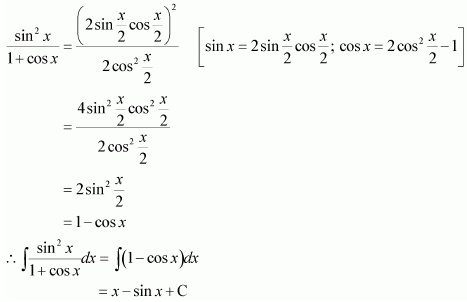Question 13.$\frac { cos2x-cos2\alpha }{ cosx-cos\alpha }$
Solution:Question 14.$\frac { cosx-sinx }{ 1+sin2x }$
Solution:Question 15.$\int { { tan }^{ 3 }2x\quad sec2x\quad dx=I }$
Solution:Question 16.
tan4x
Solution:Question 17.$\frac { { sin }^{ 3 }x+{ cos }^{ 3 }x }{ { sin }^{ 2 }x{ cos }^{ 2 }x }$
Solution: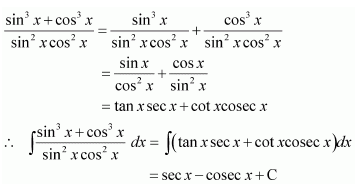Question 18.$\frac { cos2x+{ 2sin }^{ 2 }x }{ { cos }^{ 2 }x }$
Solution: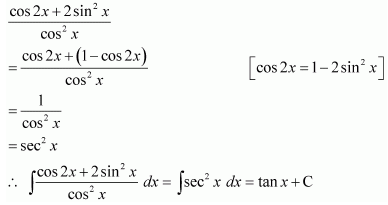Question 19.$\frac { 1 }{ sinx{ cos }^{ 3 }x }$
Solution: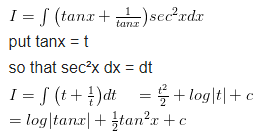Question 20.$\frac { cos2x }{ { (cosx+sinx) }^{ 2 } }$
Solution:Question 21.
sin-1 (cos x)
Solution: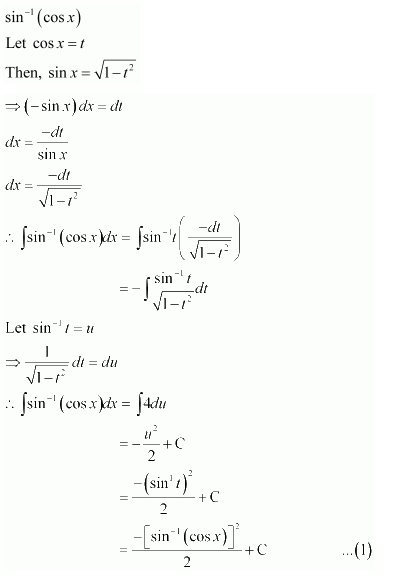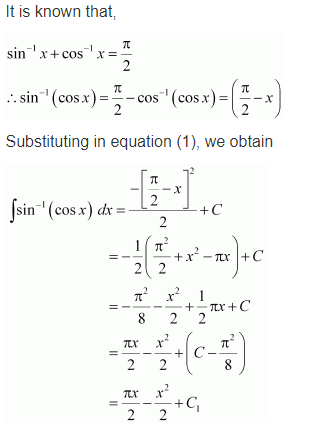Question 22.$\int { \frac { 1 }{ cos(x-a)cos(x-b) } dx }$
Solution:Question 23.$\int { \frac { { sin }^{ 2 }x-{ cos }^{ 2 }x }{ { sin }^{ 2 }x{ cos }^{ 2 }x } } dx\quad is\quad equal\quad to$
(a) tanx+cotx+c
(b) tanx+cosecx+c
(c) -tanx+cotx+c
(d) tanx+secx+c
Solution: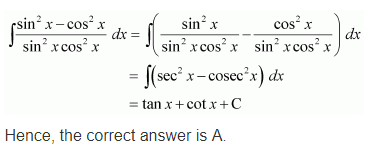Question 24.$\int { \frac { e^{ x }(1+x) }{ cos^{ 2 }({ e }^{ x }.{ x }) } } dx\quad is\quad equal\quad to$
(a) -cot(e.xx)+c
(b) tan(xex)+c
(c) tan(ex)+c
(d) cot ex+c
Solution: